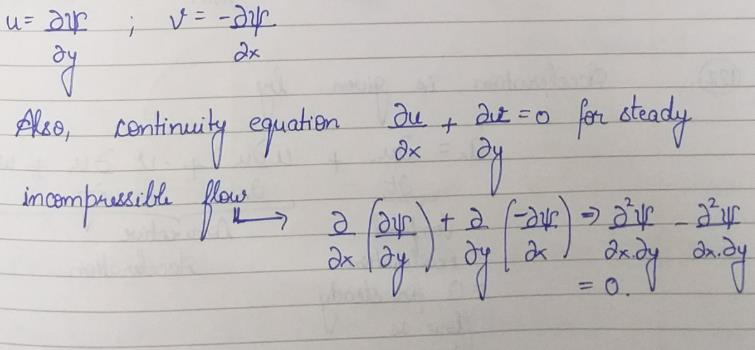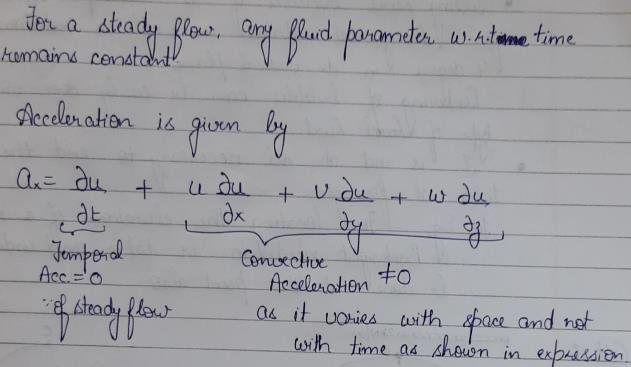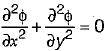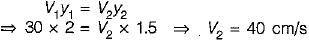Courses

# Fluid Flow Kinematics - 2

## 10 Questions MCQ Test Mock Test Series for Civil Engineering (CE) GATE 2020 | Fluid Flow Kinematics - 2

Description
This mock test of Fluid Flow Kinematics - 2 for Civil Engineering (CE) helps you for every Civil Engineering (CE) entrance exam. This contains 10 Multiple Choice Questions for Civil Engineering (CE) Fluid Flow Kinematics - 2 (mcq) to study with solutions a complete question bank. The solved questions answers in this Fluid Flow Kinematics - 2 quiz give you a good mix of easy questions and tough questions. Civil Engineering (CE) students definitely take this Fluid Flow Kinematics - 2 exercise for a better result in the exam. You can find other Fluid Flow Kinematics - 2 extra questions, long questions & short questions for Civil Engineering (CE) on EduRev as well by searching above.
QUESTION: 1

### A 2-D flow having velocity V = (x + 2y + 2)i + (4 - y)j will be

Solution:

X component of the velocity = Vx = u = x+ 2y+ 2;
y component of the velocity = Vy = v = 4-y;
z component of the velocity = Vz = w =0
Continuity Equation for the incompressible flow must be satisfied which is given by—
∂u/∂x+∂v/∂y+∂w/∂z = 0
Therefore, (∂(x+2y+2))/∂x+(∂(4-y))/∂y+(∂(0))/∂z= 1 -1 +0 = 0
Hence, the flow is incompressible.
For flow to be irrotational,
Angular Velocity = ω ⃗=1/2 (curl ( v) ⃗ )
x- component of angular velocity -> ω ⃗_z=0.5 (∂v/∂x-∂u/∂y) = 0
Since the flow is 2-d and no z- component of velocity, therefore, x and y components of the velocity will be automatically zero.

Hence, the flow is incompressible and irrotational.

QUESTION: 2

Solution:
QUESTION: 3

### A stream function is

Solution:

A stream function is defined by following characteristic:
The partial derivative of stream function w.r.t y will give velocity in x-direction.
The partial derivative of stream function w.r.t x will give velocity in negative y-direction.
It is valid for steady, incompressible flow since, is satisfies the continuity equationQUESTION: 4

The continuity equation for steady incompressible flow is expressed in vector notation as

Solution:
QUESTION: 5

In a converging steady flow there is

Solution:QUESTION: 6

In two dimensional flow, the equation of a streamline is given as

Solution:
QUESTION: 7

The concept of stream function which is based on the principle of continuity is applicable to

Solution:

Velocity potential function is valid for 3-dimensional flow while stream function is valid for 2 dimensional flow.

QUESTION: 8

Which of the following velocity potentials satisfies continuity equation?

Solution:

For the velocity potential function to satisfy continuity equation:Where φ is velocity potential, φ = x2 - y2 satisfies this equation

QUESTION: 9

In a two dimensional incompressible steady flow around an airfoil, the stream lines are 2 cm apart at a great distance from the airfoil, where the velocity is 30 m/sec. The velocity near the airfoil, where the stream lines are 1.5 cm apart, is

Solution:QUESTION: 10

The velocity potential function for a source varies with distance r as

Solution:

Velocity at any point r in the flow field of source is given by, Vr = q/2πr.